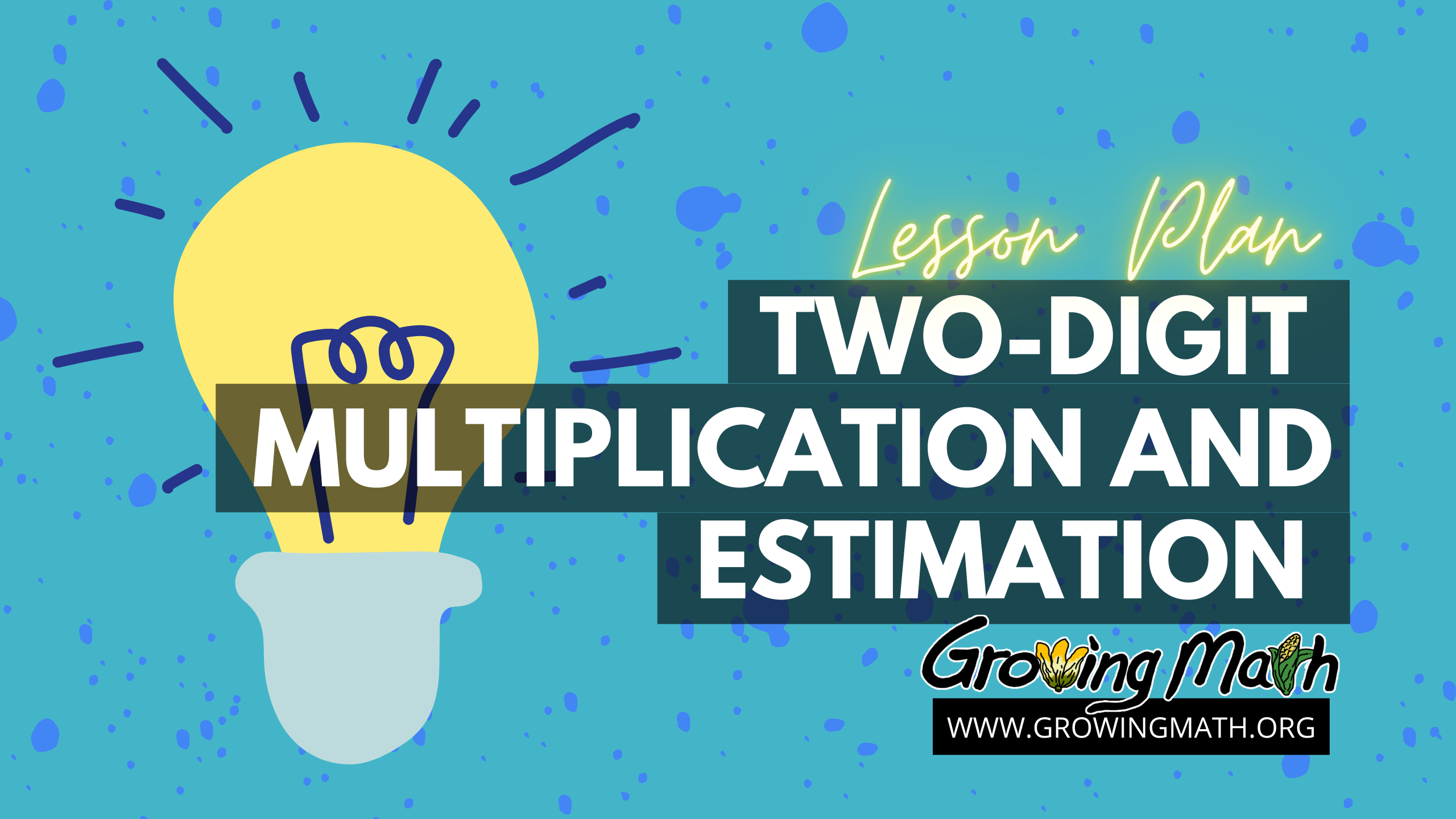# Two-Digit Multiplication and Estimation

## 📖Standard

CCSS.MATH.CONTENT.4.NBT.B.5 – Multiply a whole number of up to four digits by a one-digit whole number, and multiply two two-digit numbers, using strategies based on place value and the properties of operations.

35- 45 minutes

## 📲Technology Required

Computer with a projector, Smartboard or other device for your class to watch videos

NOTE: You’ll also need 20 math problems: 3-digit numbers multiplied by a 2-digit number. You can use what you already have, use the worksheet provided or use the Multiplication Worksheet Generator to make your own worksheet.

## 📃Lesson Summary

Students practice multiplication for a few problems, watch a video on using estimation to solve problems, hear a brief presentation from their teacher, solve more practice problems, watch a second video and practice more problems. They end the lesson with a game and a discussion of which strategies proved most useful.

## 📚Lesson Plan

#### Have the students solve 3 to 5 problems.

NOTE: This lesson requires a total of 9 to 15 multiplication problems. This worksheet has 24 problems multiplying a three-digit numbers by a two-digit number. Teachers can assign any selection of these problems or use their own. We give the students a worksheet of 24 problems and tell them to pick any ones they want to solve. Of course, teachers should do whatever works for them.

### Watch a video

This video explains steps in long multiplication, with examples and a reality check.

### Discuss the video

Use this Google slides presentation for a short discussion of the video and how to apply the information learned about estimation and long multiplication.

### Practice multiplying 2-digit and 3-digit numbers again

Now that students have had a chance to watch and discuss the video showing long multiplication, step by step, it’s time for them to practice again. They have 10 minutes to complete another 3 to 5 math problems.

### Watch another video to review the steps

Now that students have watched one video and practiced their skills with a few problems they watch a second video to reinforce those steps.

### Practice multiplying 2-digit and 3-digit numbers again

Now that students have had a chance to watch and discuss the video showing long multiplication, step by step, it’s time for them to practice again. They have 10 minutes to complete another 3 to 5 math problems.

You may wish to share the answer key with students and have them grade their own or their peer’s problems.

### Discussion

As a class, discuss strategies for solving problems, including doing a reality check, estimation and breaking problems into smaller problems. Ask students which strategies worked best for them.

### Play a Game

Both Spirit Lake: The Game and Making Camp Dakota (released in February, 2021) teach multiplication of two- and three-digit numbers.

## Assessment

In addition to grading all of the multiplication problems, remember that you can always see your students’ performance on the problems in Making Camp and Spirit Lake by accessing the reports page. You will need to enter the password you received during training.